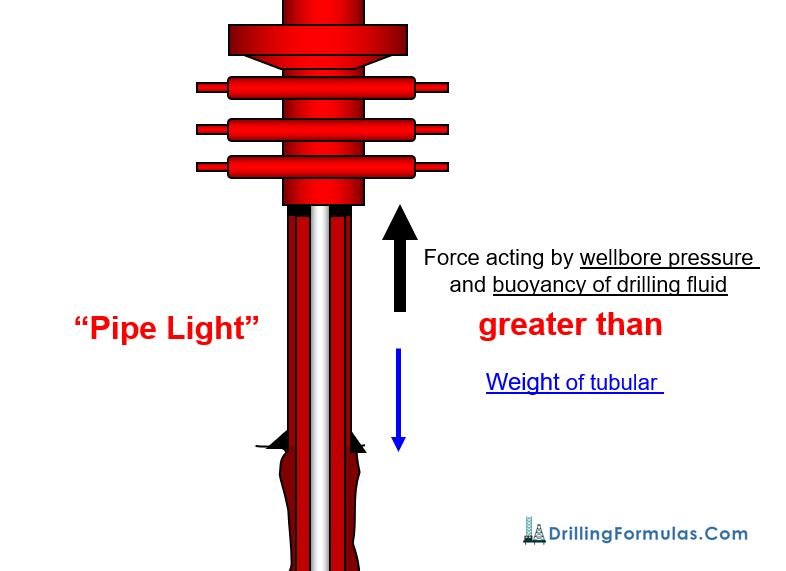# Pipe Light Calculation

Pipe light (figure 1) describes the condition of the well which well head pressure and buoyancy acting upward against a cross sectional area of pipe is greater than weight of the string. If the light pipe condition is happened and the string is not controlled by the snubbing unit, the string will be pushed out of the well.Figure 1 – Diagram for Pipe Light Condition

You may see these videos showing the pipe light condition. These videos below clearly demonstrate when the pipe is in “Pipe Light” condition. You can see that the tubing string is  hydraulically pushed out of the hole by wellbore pressure.

When workover the well under pressure with either a rig or a hydraulic workover (Figure 2), there is a risk of pipe light so it is imperative to know which condition of the well will be pipe light.

Figure 2 – Snubbing Unit (Hydraulic Workover) works against wellbore pressure. Image from RIGZONE.COM

After knowing the concept of light pipe, we will apply it to determine how many feet of tubular required snubbing into the well before the weight will overcome the surface pressure plus buoyancy force.

Basic drilling formulas for pipe light calculation are as follows;

### Buoyancy factor = (65.5 – mud weight) ÷65.5

Note: The biggest size of tubular planned to run in hole is used to determine force acted to the tubular string.

## Example

This example demonstrates how to determine light of the tubing string at the pipe light condition.

For this example, we will use the oilfield units where;

• Force in lb
• Cross sectional area in square inch
• Diameter in inch
• Mud weight in ppg

Wellbore Information

Surface pressure = 800 psi

Brine weight in hole = 9.0 ppg

Tubing size = 2 -7/8”, 6.4 ppf

Disregard friction for the calculation## Solution

Cross sectional area of tubing = 0.7854 x 2.8752 = 6.492 square inch

Force of wellbore pushing = 6.492 x 800 = 5,194 lb

Buoyancy factor = (65.5 – 9) ÷ 65.5 = 0.8626

Tubing must have a total weight of 5,194 lb to overcome the wellhead pressure however we need to account for buoyancy factor because this factor reduces the weight. Therefore, the total weight accounted for buoyancy factor is equal to calculated weight in the air divided by buoyancy factor.

Total tubing weight accounted for buoyancy factor = 5,194 ÷0.8626 =6,021 lb.

Total length of tubing (ft) = Total weight ÷ weight per ft

Total length of tubing (ft) = 6,021 ÷ 6.4 = 941 ft

If the tubing length is less than 941 ft, this will be under “Pipe Light” condition and the string will be hydraulically pushed out of hole.

References

Azar, J. and Samuel, G. (2008). Drilling engineering. 1st ed. Tulsa, Okla.: PennWell.

Bourgoyne, J., Millheim, ‎., Chenevert, M. and Young, ‎. (1986). SPE Textbook Series, Volume 2 : Applied Drilling Engineering. 1st ed. Society of Petroleum Engineers.

Mitchell, R., Miska, S. and Aadny, B. (2011). Fundamentals of drilling engineering. Richardson, TX: Society of Petroleum Engineers.

Share the joy